# ISEE Middle Level Math : How to find the area of a parallelogram

## Example Questions

### Example Question #1 : How To Find The Area Of A Parallelogram

Find the area of the following parallelogram: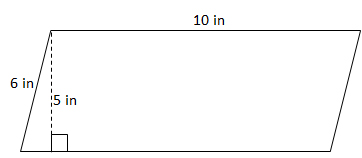Note: The formula for the area of a parallelogram is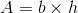.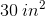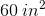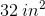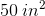Explanation:

The base of the parallelogram is 10, while the height is 5.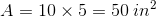### Example Question #2 : How To Find The Area Of A Parallelogram

Find the area: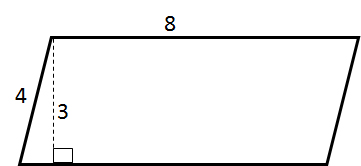Explanation:

The area of a parallelogram can be determined using the following equation: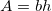Therefore,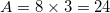### Example Question #3 : How To Find The Area Of A Parallelogram

You can solve the area of a parallelogram when you know the lengths of each of the sides. True or False?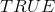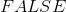Explanation:

The area of a parallelogram is found by computing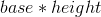.  In this situation, you would have the base which is the bottom side but you would not have the height measurement.  Since you would not be able to solve for the area with just the side lengths, the statement is.

### Example Question #4 : How To Find The Area Of A Parallelogram

If a parallelogram has side lengths ofand, what is the area?Cannot be determined.Cannot be determined.

Explanation:

To find the area of a parallelogram, you use the formula,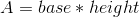.

Since the height in this problem is not known, you cannot solve for area.

### Example Question #5 : How To Find The Area Of A Parallelogram

Find the area of a parallelogram with a base of 6 inches and a height of 9 inches.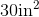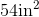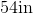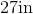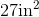Explanation:

To find the area of a parallelogram, we will use the following formula: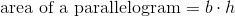where is the base and h is the height of the parallelogram.

Now, we know the base has a length of 6 inches. We also know it has a height of 9 inches.  Knowing this, we can substitute into the formula.  We get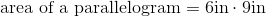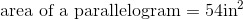### Example Question #6 : How To Find The Area Of A Parallelogram

Find the area of the parallelogram with a base length of 6 and a height of 15.Explanation:

Write the area formula of a parallelogram.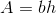Substitute the dimensions into the formula.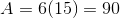The answer is: